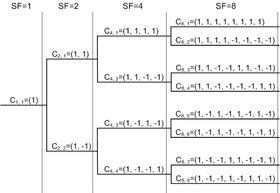# Chip (CDMA)

﻿
Chip (CDMA)

In digital communications, a chip is a pulse of a direct-sequence spread spectrum (DSSS) code, such as a pseudo-noise code sequence used in direct-sequence code division multiple access (CDMA) channel access techniques.

In a binary direct-sequence system, each chip is typically a rectangular pulse of +1 or –1 amplitude, which is multiplied by a data sequence (similarly +1 or –1 representing the message bits) and by a carrier waveform to make the transmitted signal. The chips are therefore just the bit sequence out of the code generator; they are called chips to avoid confusing them with message bits.

The chip rate of a code is the number of pulses per second (chips per second) at which the code is transmitted (or received). The chip rate is larger than the symbol rate, meaning that one symbol is represented by multiple chips. The ratio is known as the spreading factor (SF) or processing gain:$\ \mbox{SF} = \frac{\mbox{chip rate}}{\mbox{symbol rate}}$